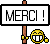[Livre] Optimization for Data Analysis
Optimization for Data*Analysis

Optimization techniques are at the core of data science, including data analysis and machine learning. An understanding of basic optimization techniques and their fundamental properties provides important grounding for students, researchers, and practitioners in these areas. This text covers the fundamentals of optimization algorithms in a compact, self-contained way, focusing on the techniques most relevant to data science.

An introductory chapter demonstrates that many standard problems in data science can be formulated as optimization problems. Next, many fundamental methods in optimization are described and analyzed, including: gradient and accelerated gradient methods for unconstrained optimization of smooth (especially convex) functions; the stochastic gradient method, a workhorse algorithm in machine learning; the coordinate descent approach; several key algorithms for constrained optimization problems; algorithms for minimizing nonsmooth functions arising in data science; foundations of the analysis of nonsmooth functions and optimization duality; and the back-propagation approach, relevant to neural networks.

[Lire la suite]